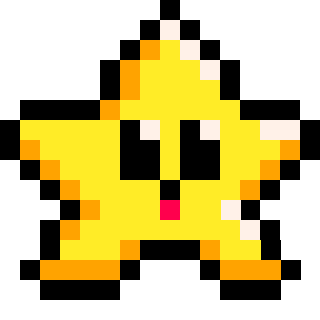# BOOL

A boolean (often shortened to "bool") is a type of variable that can have one of two values: true or false.

``bool = true``

It is like a light switch that has only two states: on or off. The term "boolean" is named after George Boole, a 19th-century English mathematician and logician.

Boolean variables are used in programming to represent logical states or conditions. For example, you might use a boolean variable to check if a certain condition is true or false, and then make a decision based on that condition.

``````coins = 0
win = false          --set boolean

if coins > 10 then
win = true         --change boolean
end

if win==true then    --check boolean
print("you win!")
end
``````

#### Easy Toggle Switch!

``bool = not bool``

This is an easy way to flip a boolean variable to true if it is false and back to false if it is true. It uses the not operator to mean the opposite value. This is useful for any kind of toggling that you want to do whether controlled by a player's button press or by a trigger in the game.

Example:

``````toggle = false

--longform
if btnp(4) then
if toggle==true then
toggle=false
else
toggle=true
end
end

--shorthand
if (btnp(4)) toggle = not toggle``````

The above "shorthand" example of toggling a boolean uses the shorthand if statement and the not operator. This does save on both characters and tokens.

To explain the shorthand single line of code, it first checks if the player presses button #4, and every time they do, then it sets the variable named "toggle" to the opposite value of what it already is. So if toggle is true then it will be set to not true (false), and if it is already false, then it will set to not false (true).284

19 May 2023

`Font`